• ## 分治法详解二维最近点对问题

千次阅读 多人点赞 2020-04-14 16:47:31
算法设计与分析——分治：详解二维最近点对问题1 前言2 问题描述3 分治4 暴力求解4.1 算法思路4.2 时间复杂度分析4.3 代码实现5 分治求解5.1 算法思路5.1.1 数据预处理5.1.2 划分中轴线5.1.3 求半边最小距离...


目录
1 前言2 问题描述3 分治法4 暴力求解4.1 算法思路4.2 时间复杂度分析4.3 代码实现
5 分治法求解5.1 算法思路5.1.1 数据预处理5.1.2 划分中轴线5.1.3 求半边最小距离5.1.4 求中间的最小距离
5.2 代码实现5.2.1 数据预处理5.2.2 分治法

6 执行结果

1 前言
转载请注明出处。
2 问题描述
问题描述：二维平面上有n个点，如何找到距离最近的点？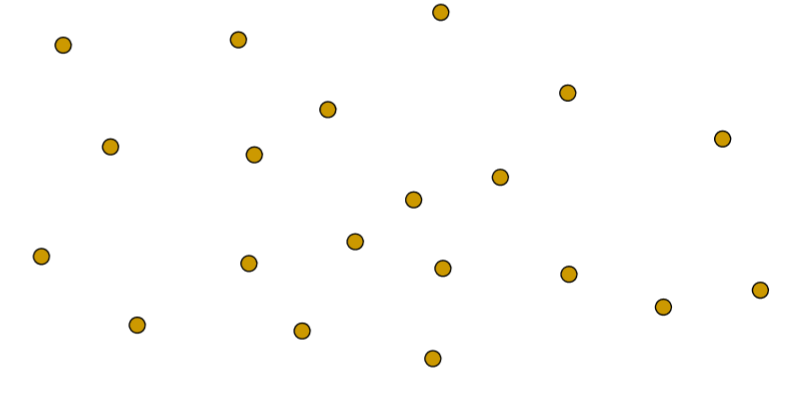3 分治法

分治法：将一个规模较大的问题分解为规模较小的子问题，先求解这些子问题，然后将各子问题的解合并得到原问题的解的思路。 递归：直接或间接地调用自身方法。递归通常是解决分治的办法。
递归缺点：具体执行步骤理解比较复杂；递归优点：易于实现，大体思路清晰。

4 暴力求解
4.1 算法思路
二维平面上有n个点，则有(n-1)+(n-1)+...+1=n(n-1)/2个点对，依次遍历求出所有点对的距离即可。
4.2 时间复杂度分析
由4.1分析可知，即总共要求出n(n-1)/2个距离，故时间复杂度O(n^2)
4.3 代码实现
	public static double getByBruteFoce(double[][] points) {
int tail=points.length-1;
for(int j=i+1;j<=tail;j++) {
if(getDistance(points,i,j)<minDist) {
minDist=getDistance(points,i,j);//更新点对最小距离
minIndex=i;//更新点对序号
minIndex=j;
}
}
}
return minDist;
}

5 分治法求解
5.1 算法思路
核心思想：
数据预处理划分中轴线求出左边的最小距离求出右半边的最小距离求出中间的最小距离比较这三个最小距离
5.1.1 数据预处理
因为这些点的位置是随机产生并保存在二维数组中，所以我们得先将这些点，按照x坐标从小到大排序，调整它们在二维数组中的次序。比如：最左边的点，它的位置就保存在二维数组的第一个元素中；
5.1.2 划分中轴线
把这些点在平面上分成左右两边。 依据什么来划分中轴线？ 选择最中间的两个元素，求出它俩x坐标的平均值，设置为中轴线的坐标。
5.1.3 求半边最小距离
左半边和右半边的求最小距离的方法是一样的。 假如我们现在求的是左半边，那就把左半边也看成一个整体，我们再把它分成左右两半，依次往下递归，越分越小。 递归何时中止？ 分到点只剩很小的时候就终止，比如本文在后面附加的代码中，是当平面只剩下4个点时就不再切分。
5.1.4 求中间的最小距离
中间区域应该划分多宽？ 我们只需要考查中轴线左右两边距离小于d的点。 理由：距离中轴线大于d的那些点，它们和另一个半边的点的距离，肯定大于d，考查他们就没有意义了。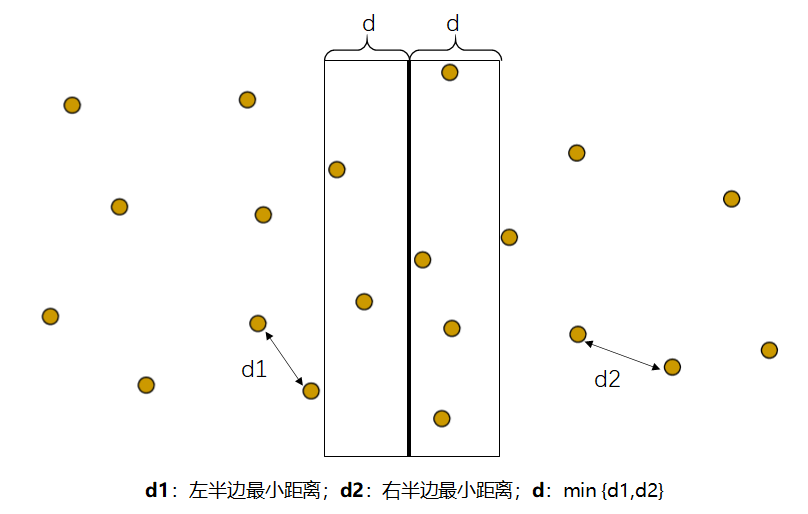那么，现在我们只需要对上图的左侧2个点和右侧4个点分别进行比较即可。
如何进一步优化？ 比如我们现在选取了左侧的m点，但实际上，我们不必跟右侧的4个点都比较，只需跟右侧的阴影部分(d*2d)以内的点进行比较，也就是，跟m点垂直距离在d以内的那两个点。 原因：如果两点之间垂直距离大于d，那么这两点间距必然大于d，考查他们也没有意义。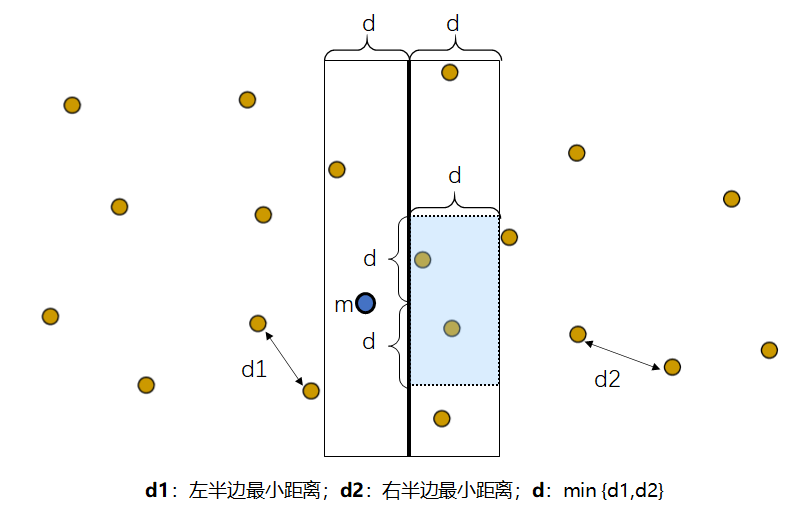关于矩形的进一步说明 如果查阅过其他资料，或许你听过，我们划分出的d*2d区域中，最多只能有6个点。 因为我们已知两侧的最小距离为d，而d*2d的区域中的点是同一侧的。因此这些点的距离必然大于等于d，经过数学几何知识，我们可以算出这个区域最多只能有6个点。
5.2 代码实现
5.2.1 数据预处理
在这里，我是采用了效率较高的快速排序算法。
	//数据预处理：将点按照横坐标由小到大排序
//快速排序
private static double[][] quickSort(double[][] oldData) {
double[][] newData=oldData.clone();
QSRecursion(newData,0,newData.length-1);
return newData;
}
private static void QSRecursion(double[][] newData, int i, int j) {
if(i==j)return;
int standardIndex=i+new java.util.Random().nextInt(j-i+1);
int pivot=partition(newData,i,j,standardIndex);//保存基准元素的正确位置
if(pivot!=i)QSRecursion(newData,i,pivot-1);//左边
if(pivot!=j)QSRecursion(newData,pivot+1,j);//右边
}
private static int partition(double[][] newData, int i, int j, int standardIndex) {
swap(newData,i,standardIndex);//将序列首个元素和基准元素对换位置
int left=i+1;//i为头指针
int right=j;//j为尾指针
while(left<right) {
while(newData[left]<=newData[i]&left<right) {
left++;
}
while(newData[right]>=newData[i]&left<right) {
right--;
}
if(left<right) {
swap(newData,right,left);//对换位置,保证左小右大
}
}
if(newData[right]>newData[i]) {
swap(newData,right-1,i);
standardIndex=right-1;
}else {
swap(newData,j,i);
standardIndex=j;
}
return standardIndex;
}
private static void swap(double[][] newData, int a, int b) {//对换位置
double[] temp=newData[a];
newData[a]=newData[b];
newData[b]=temp;
}

5.2.2 分治法
	//分治法求解
public static double getByDivideConquer(double[][] points) {
if(points.length==1|points.length==0) {
return 0;
}
double[][] pointsSorted=quickSort(points);
//		for(int i=0;i<pointsSorted.length;i++)//检查排序是否正确
//		{
//			      System.out.println(pointsSorted[i]);
//
//		}
int tail=pointsSorted.length-1;
double minDist=getDistance(pointsSorted,minIndex,minIndex);
return minDist;
}
/
private static int[] getByDivideConquer(double[][] points, int head, int tail) {
for(int j=i+1;j<=tail;j++) {
if(getDistance(points,i,j)<minDist) {
minDist=getDistance(points,i,j);
minIndex=i;
minIndex=j;
}
}
}
}else {
double minDisLeft=getDistance(points,minIndexLeft,minIndexLeft);
double minDisRight=getDistance(points,minIndexRight,minIndexRight);
double minDisTwoSide=Math.min(minDisLeft, minDisRight);//即d
if(minDisLeft>=minDisRight) {
minDist=minDisRight;
minIndex=minIndexRight;
}else {
minDist=minDisLeft;
minIndex=minIndexLeft;
}
while(i<=tail&&(points[i]-middleAxis<minDisTwoSide)) {//i点没越界且和中间线的x轴距离小于d
{
int count=0;
if(Math.abs(points[j]-points[j])<minDisTwoSide) {//找出d*2d矩形的点
if(getDistance(points,i,j)<minDist) {
minDist=getDistance(points,i,j);
minIndex=i;
minIndex=j;
}
count++;
}
}
i++;
}
}
}
return minIndex ;
}
//
//计算点i和点j的距离
private static double getDistance(double[][] points, int i, int j) {
double dis=Math.sqrt(Math.pow(points[i]-points[j], 2)+Math.pow(points[i]-points[j], 2));
//System.out.println(dis);//测试输出
return dis;
}

6 执行结果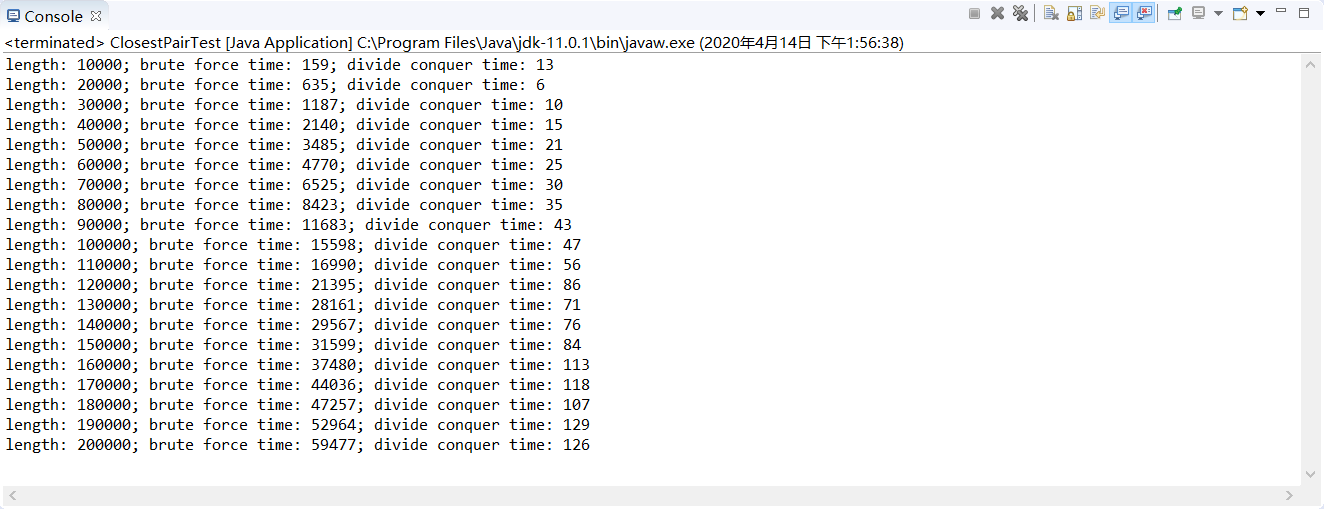展开全文算法 递归 快速排序
• 目录写在前面问题描述求解问题的算法原理描述分治分治的改进：算法核心伪代码测试流程算法测试结果及效率分析总览与对比分治：特殊情况的测试图形演示求解这个问题的经验总结代码最近点对图形演示 ...
实验代码 + 报告资源：
链接: https://pan.baidu.com/s/1CuuB07rRFh7vGQnGpud_vg
提取码: ccuq


目录
写在前面问题描述求解问题的算法原理描述分治法分治法的改进：
算法核心伪代码测试流程算法测试结果及效率分析总览与对比分治法：特殊情况的测试
图形演示对求解这个问题的经验总结代码最近点对图形演示

写在前面
期末终于算法课快要完结了。
这学期算法课可谓是最难顶的课程了，又正好是线上上课，提问互动的机会相对较少，老师上课抛砖引玉，实验内容又比较难，我花了大部分的时间在找算法，实现算法，改算法bug上。
我也参考过很多往届师兄的报告，但是大多都比较抽象晦涩，而且没有代码只讲方法，比较难以理解具体实现的细节。
所以我打算记录一下自己的报告+代码，前人coding后人copying ，希望让大家少走弯路。。。
注意：不要直接copy代码，这是冲塔行为！查重系统鲨疯辣。
问题描述
对于平面上给定的N个点，给出所有点对的最短距离，即，输入是平面上的N个点，输出是N点中具有最短距离的两点。  要求随机生成N个点的平面坐标，应用蛮力法编程计算出所有点对的最短距离。  要求随机生成N个点的平面坐标，应用分治法编程计算出所有点对的最短距离。  分别对N=100000—1000000，统计算法运行时间，比较理论效率与实测效率的差异，同时对蛮力法和分治法的算法效率进行分析和比较。  如果能将算法执行过程利用图形界面输出，可获加分。
求解问题的算法原理描述
暴力法： 暴力法的思路相对简单，即枚举所有可能的配对情况，然后一一比对，找到距离最小的点，一共有 Cn2 种组合，也就是 n*(n-1)/2种，总的时间复杂度是O(n^2)
暴力法伪代码：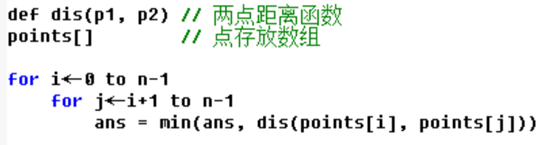分治法
问题分割策略：排序，取中位数
如果像快速排序，分割的策略与数据有关，那么算法便会退化，不能达到稳定的O(nlogn)
但是如果效仿归并排序，每次选取中点进行分割，那么可以使得递归树的深度稳定的控制在log（n）
这里对点做预处理，按照x升序，x相同则y升序排序，这样我们每次选取中间下标的点，都能尽量地将点分割成几何上的两半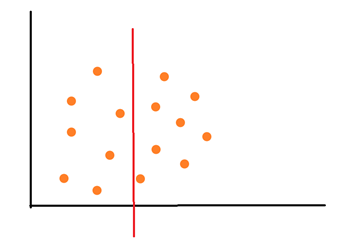子问题存在分析： 问题是选取两个点，p1，p2，使得他们距离最短，子问题存在于以下空间
p1 p2 同时位于左边点集p1 p2 同时位于右边点集p1 p2 一个在左侧一个在右侧，对于子问题1和2，我们可以通过递归直接解决，难点就在于解决子问题3，即两点在两边的情况
子问题3求解 缩小空间：
想起来归并是要【合并】的，如何有效利用递归得到的答案呢？ 如果要一一配对找两边点求距离，那么合并效率还是O(n^2)
递归得到两边点子集的最近点对距离，取最小值d，我们可以借此距离d来缩小我们查找的空间
从中间点向外扩散，如果点与中间点的x左边之差，大于d，那么这个点及其往后的点，都不可能是最优的答案，缩小了查找空间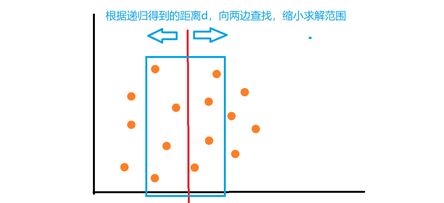缩小查找空间，其实是不稳定的，可能查找空间和原来一样，所以我们不能暴力枚举查找空间内的点，下面引入一个结论，来帮助我们快速查找
所以引入一个结论来帮助求解：

结论：对于左边区域的每一点p，要想在右边区域找到一点p’使得pp’距离<=d，这样的点p’必定存在于【以p的y坐标为中心，上下延展d形成的d*2d的子矩形中】

证明：假设极限情况，p在左右边线上，以p为中心半径为d画圆，半圆区域内，都是距p的距离小于d的，半圆是矩形的真子集，故目标点一定存在于矩形中 而且因为右侧的点具有稀疏性，即两两之间距离大于等于d， d*2d的矩形区域，矩形区域内最多存在6个点（如果圆形区域则是四个）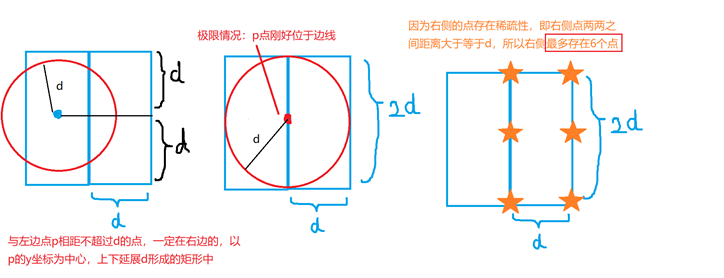我们遍历左边区域的所有点，划分出d2d的矩形区域，然后检查6个点，更新答案即可，可是如何用短时间找到d2d的矩形区域？
对左右的子区域的所有点按照y升序排序（左右分开排序，不是混合在一起），在指针i升序遍历左边的所有点的时候，设置指针h在右边的点中向后查找，找到第一个不矮于i点y坐标d高度的点h（即h和i点高度差小于d），从h往后找6个点即可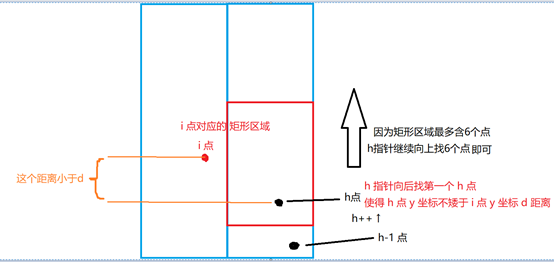因为左右点集都是升序，这表明，i++之后，下一个i点的d*2d矩形的下边界一定会提升，而h指针随着做出更新即可
因为i点矩形的下边界是递增的，h指针不走回头路，h指针最多从0增加到右边区域点的个数，最多n/2次
所以遍历左边点集，h迭代的次数，均摊到每一次i迭代，就是均摊一次，O(1)，而查找矩形区域总共是6个点，也是常数时间可以完成，所以总的复杂度仍然是O(n)
分治法的改进：
因为每次递归之后，还要对左右按d划分的区域排序，总复杂度是O(2*n/2log(n/2))， 即 O(nlog(n/2))，合并代价，不是线性效率
效仿归并排序，每次二分递归之后，我们对数组归并，因为递归排好了左右子区间，这样归并后，数组就是对y有序的了，不用消耗额外的时间再排序了，可以使合并子问题的代价变为O(n)级别
算法核心伪代码
暴力法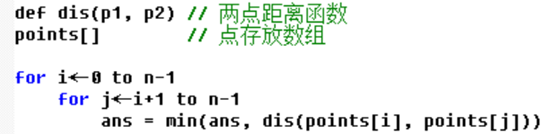单纯分治法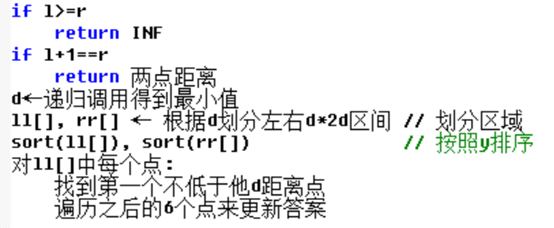递归+归并法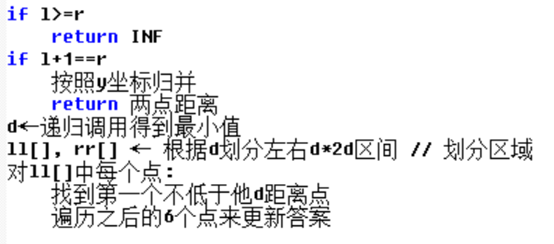测试流程
测试之前做了270万（2715633）次验证，生成随机数据，比对两种分治法与暴力法的结果（暴力法保证正确），结果三种方法结果全部相等，排除算法bug导致时间误差
每一次测试随机生成50组规模为batch的数据，利用哈希set确保数据中没有重复的点，点坐标的范围是 [0，sqrt(batch)*3] 保证随着规模的变化，数据的密集程度不会有太大的改变，排除点稀疏程度导致的时间误差
生成的每组数据被复制若干份，保证每次每个算法用到的数据是同一批，排除数据随机生成导致的时间误差
算法测试结果及效率分析
暴力法：显而易见时间复杂度为O(n^2)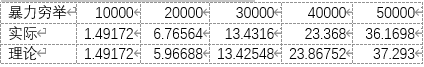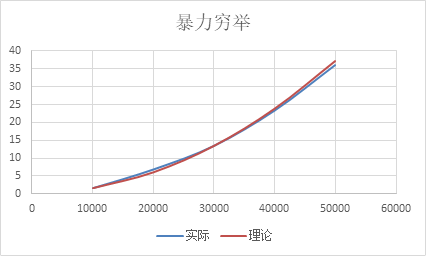从图像上，图像符合n^2函数的趋势 数据上，规模扩大k倍时，时间开销扩大k^2倍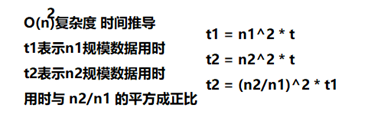单纯的分治法：如果我们直接在合并的时候排序，那么相当于合并代价是nlog(n)
根据递推法，我们可以很快得到，单纯的分治法，递推式如下：T(n) = 2T(2/n) + nlog(n)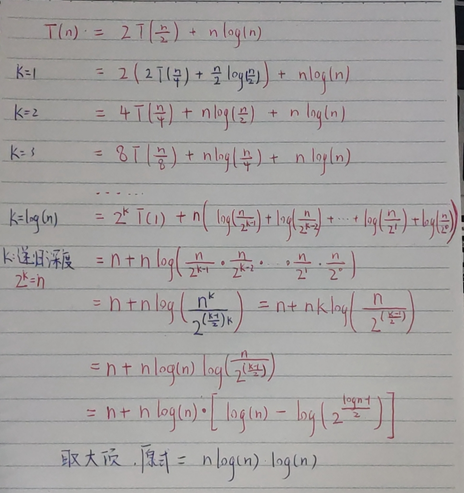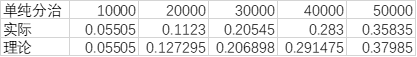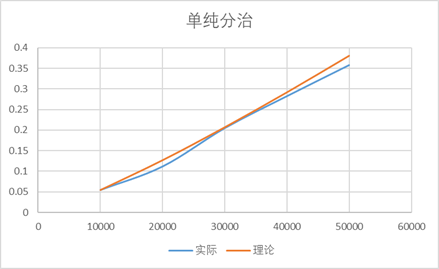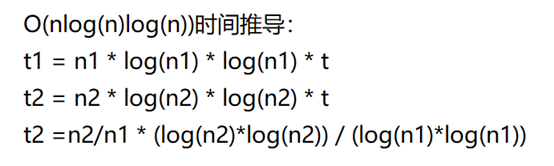图像上基本符合二次增长，而时间上也是符合上述的推导
增大测试规模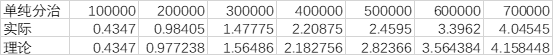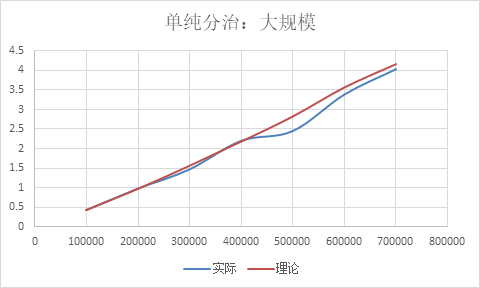归并+分治： 效仿归并排序，如果我们在分治的同时按照y升序进行归并排序操作，递归后有序，不需要额外在递归中调用排序，合并代价从O(nlog(n))变为O(n)，总体时间复杂度变为O(nlog(n))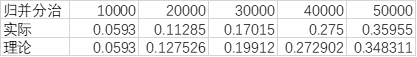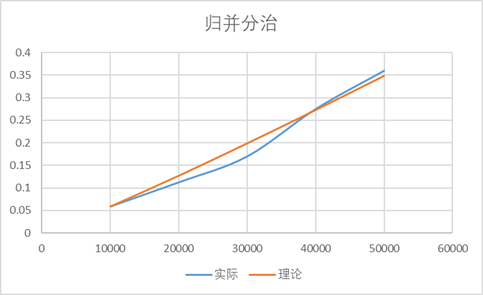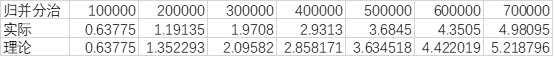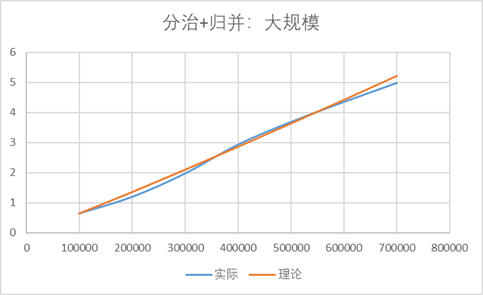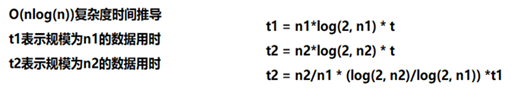在图像上基本符合二次增长，而时间上也是符合上述的推导，因为每次需要遍历数组划分d*2d的区间，导致合并代价稳定在O(n)，总体看来曲线比较稳定
总览与对比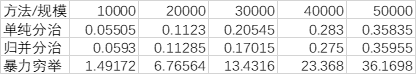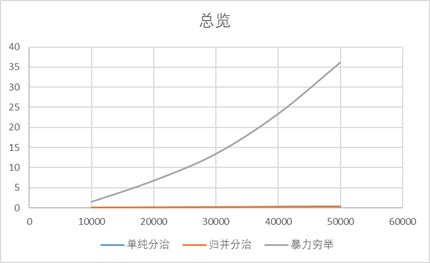可以看到不论是单纯的分治还是归并的分治，效率都要远远高于暴力法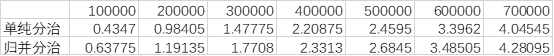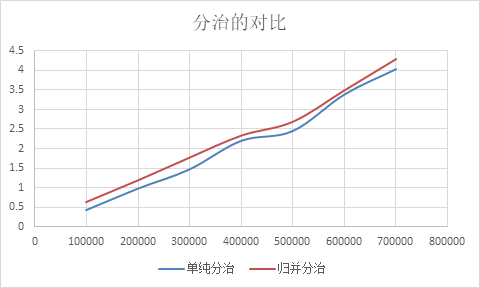结论：可以看到虽然时间复杂度不同，但是两种方法消耗的时间相差无几，可能是log(n)在十万级别规模时，带来的影响很小，而且数据也存在随机性
分治法：特殊情况的测试
使用相邻点等距离的密集点来做数据，测量结果如下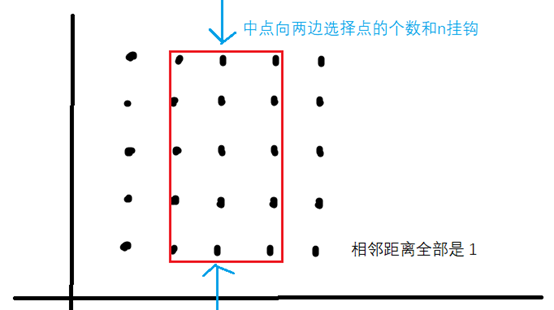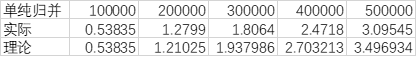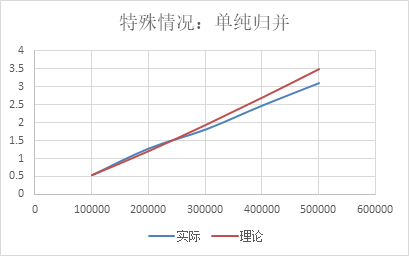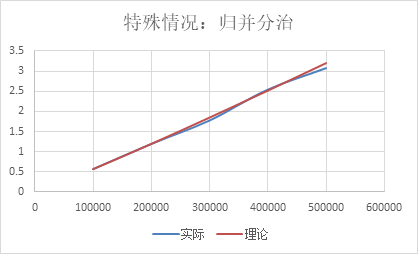因为不论是归并还是分支+归并，因为合并代价都比较稳定，在极端的数据情况下，仍然能有好的表现
图形演示
通过python的matplotlib库的pyplot子库的animation对象的子对象ArtistAnimation进行图形的绘制，在递归的同时记录栈状态，递归结束之后使用图形的方式输出递归的过程，比如边界的划分，子问题的合并，以及点的选择
Word放动图不会动，更加详细的信息请老师查看我的实验课演示，或者移步至我的GitHub查看详细的原理与演示 https://github.com/AKGWSB/graphic-demo-of-closest-point-pair-algorithm-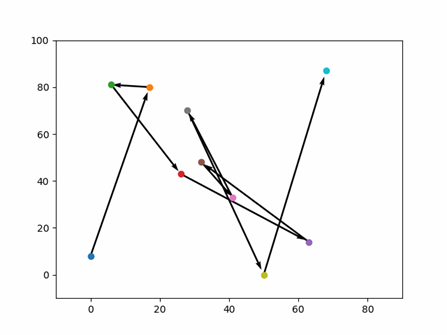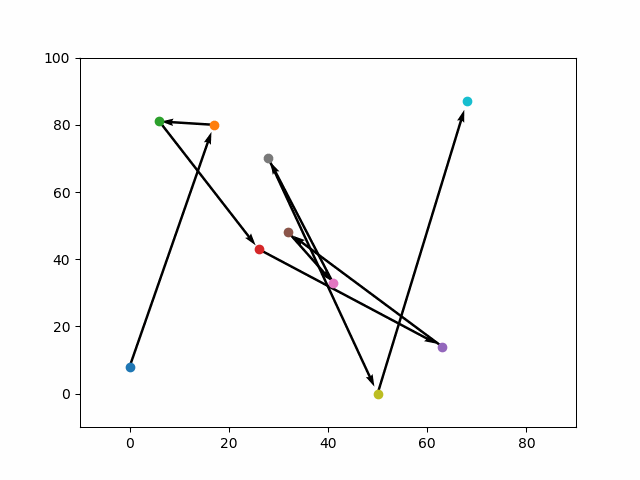对求解这个问题的经验总结
点的坐标是int存储的，而距离则是double，因为二进制存储规则不同，两者之间的传递一定要加上强制类型转化，否则容易出现截断或者是转换不当等其他异常情况
在测试递归程序的时候，一定要保证合并操作的正确性，递归的答案可以先用暴力法代替，然后观察合并的结果是否和暴力法结果一致
在测试开销和递归结果有关的递归程序的时候，一定要大量测试保证代码的正确性，再测试时间，我之前代码有bug的时候，递归算出来的d不是最小的，测量的结果受影响
遇到问题的时候要想，能否通过数学的方法，压缩问题的解，使得求解范围缩小，甚至变为常数，比如通过划分d*2d的矩形来压缩求解的空间
遇到一些分治递归的问题的时候，合并问题的时候需要考虑：如果左右已经有序了，会不会减少合并的代价？比如逆序对问题，也是通过同样的思想，分治的同时归并，然后利用数据的有序性，将合并问题的代价由O(n^2)减少到O(n)
代码的常数开销也很重要，代码常数开销过大，可能会导致预测的时间出现偏差，编程时应该尽量减小常数的开销
代码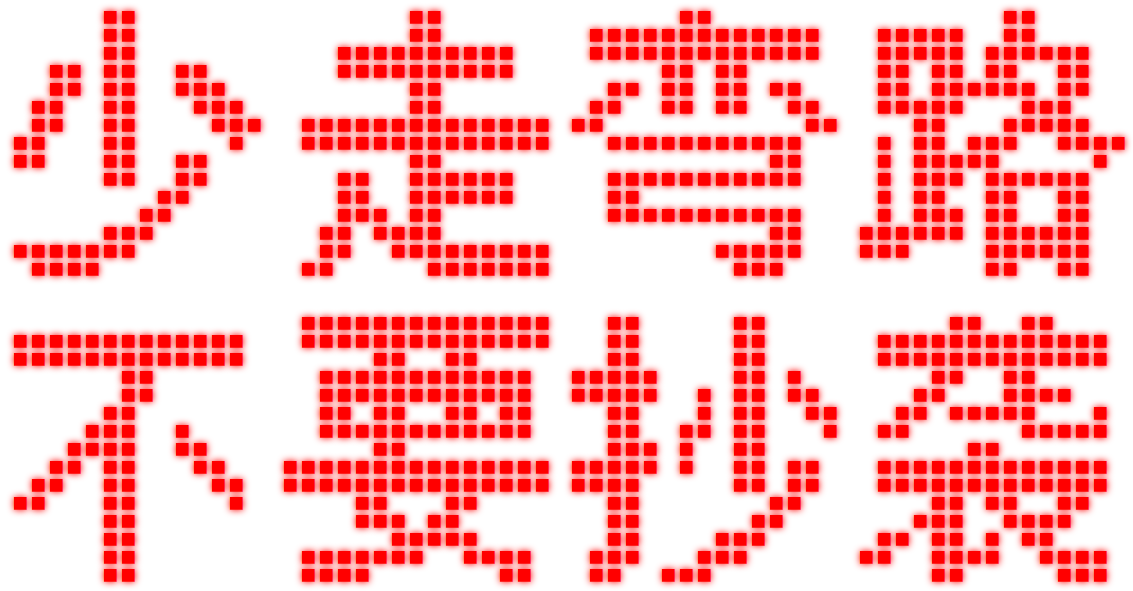最近点对
#include <bits/stdc++.h>

using namespace std;

typedef struct p
{
int x, y;
p(){}
p(int X, int Y):x(X),y(Y){}
}p;

/*
浮点最小函数，防止默认min的int形参截断
*/
double lfmin(double a, double b)
{
return (a<b)?(a):(b);
}

/*
比较函数，排序用，x升序，x相同则y升序
param p1 : 第一个点
param p2 : 第二个点
return   : p1 前于 p2 ?
*/
bool cmpx(const p& p1, const p& p2)
{
if(p1.x==p2.x) return p1.y<p2.y;
return p1.x<p2.x;
}

/*
比较函数，排序用，则y升序，归并用
param p1 : 第一个点
param p2 : 第二个点
return   : p1 前于 p2 ?
*/
bool cmpy(const p& p1, const p& p2)
{
return p1.y<p2.y;
}

/*
求解两点欧氏距离
param p1 : 第一个点
param p2 : 第二个点
return   : 距离，浮点数
*/
double dis(const p& p1, const p& p2)
{
return sqrt((double)(p1.x-p2.x)*(p1.x-p2.x)+(double)(p1.y-p2.y)*(p1.y-p2.y));
}

/*
求两点水平距离
param p1 : 第一个点
param p2 : 第二个点
return   : 水平距离，浮点数
*/
double disX(const p& p1, const p& p2)
{
double ans = (double)p1.x - (double)p2.x;
if(ans<0) return ans*-1;
return ans;
}

/*
重载哈希函数，哈希set去重复点用
param p : 点
return  : 根据点坐标得到的哈希值
*/
struct hashfunc
{
size_t operator()(const p& P) const
{
return size_t(P.x*114514 + P.y);
}
};

/*
重载比较函数，哈希set去重复点用
param p1 : 第一个点
param p2 : 第二个点
return  : 两点是否相同
*/
struct eqfunc
{
bool operator()(const p& p1, const p& p2) const
{
return ((p1.x==p2.x)&&(p1.y==p2.y));
}
};

/*
暴力求解最近点对
param points : 点的数组
return       : 最近点对距离
*/
double cp(vector<p>& points)
{
double ans = (double)INT_MAX;
for(int i=0; i<points.size(); i++)
for(int j=i+1; j<points.size(); j++)
ans = lfmin(ans, dis(points[i], points[j]));
return ans;
}

/*
分治求解最近点对，复杂度O(nlog(n)log(n))
param points : 点的数组
param l      : 点数组左端点
param r      : 点数组右端点
return       : 最近点对距离
explain      : 区间[l, r]左闭右闭
*/
double cp(vector<p>& points, int l, int r)
{
if(l>=r) return (double)INT_MAX;
if(l+1==r) return dis(points[l], points[r]);
int mid=(l+r)/2, le=mid, ri=mid, h=0;
double d=lfmin(cp(points, l, mid), cp(points, mid+1, r)), ans=d;
vector<p> ll, rr;
while(le>=l && disX(points[mid], points[le])<=d) ll.push_back(points[le]), le--;
while(ri<=r && disX(points[mid], points[ri])<=d) rr.push_back(points[ri]), ri++;
sort(ll.begin(), ll.end(), cmpy), sort(rr.begin(), rr.end(), cmpy);
for(int i=0; i<ll.size(); i++)
{
while(h<rr.size() && rr[h].y+d<ll[i].y) h++; h=min((int)rr.size(), h);
for(int j=h; j<min((int)rr.size(), h+6); j++)
if(!eqfunc()(ll[i], rr[j])) ans=lfmin(ans, dis(ll[i], rr[j]));
}
return lfmin(ans, d);
}

/*
分治+归并求解最近点对，复杂度O(nlog(n))
param points : 点的数组
param l      : 点数组左端点
param r      : 点数组右端点
return       : 最近点对距离
explain      : 区间[l, r]左闭右闭
*/
double cpm(vector<p>& points, int l, int r)
{
if(l>=r) return (double)INT_MAX;
if(l+1==r)
{
if(cmpy(points[r], points[l])) swap(points[l], points[r]);
return dis(points[l], points[r]);
}
int mid=(l+r)/2, le=mid, ri=mid, h=0;
p midp = points[mid];
double d=lfmin(cpm(points, l, mid), cpm(points, mid+1, r)), ans=d;
inplace_merge(points.begin()+l, points.begin()+mid+1, points.begin()+r+1, cmpy);
vector<p> ll, rr;
for(int i=l; i<=r; i++)
{
if(midp.x>=points[i].x && disX(midp, points[i])<=d) ll.push_back(points[i]);
if(midp.x<=points[i].x && disX(midp, points[i])<=d) rr.push_back(points[i]);
}
for(int i=0; i<ll.size(); i++)
{
while(h<rr.size() && rr[h].y+d<ll[i].y) h++; h=min((int)rr.size(), h);
for(int j=h; j<min((int)rr.size(), h+6); j++)
if(!eqfunc()(ll[i], rr[j])) ans=lfmin(ans, dis(ll[i], rr[j]));
}
return lfmin(ans, d);
}

int main()
{
// 特殊情况测试
int n,x,y,t,cnt=0;
double t1=0, t2=0, t3=0;
#define sample 20
#define batch 500000
#define lower 0
#define upper (int)sqrt(batch)+1
t = sample;
n = batch;
vector<p>  pp(n), p1, p2, p3;
for(int i=0; i<upper&&cnt<n; i++)
for(int j=0; j<upper&&cnt<n; j++)
pp[cnt].x=i, pp[cnt++].y=j;

while(t--)
{
cout<<t<<endl;
p1=pp; p2=pp;
clock_t st, ed;
double ans1, ans2, ans3;

sort(p1.begin(), p1.end(), cmpx);
st = clock();
ans1 = cp(p1, 0, p1.size()-1);
ed = clock();
t1 += (double)(ed - st) / CLOCKS_PER_SEC;
cout<<(double)(ed - st) / CLOCKS_PER_SEC<<endl;

sort(p2.begin(), p2.end(), cmpx);
st = clock();
ans2 = cpm(p2, 0, p2.size()-1);
ed = clock();
t2 += (double)(ed - st) / CLOCKS_PER_SEC;
cout<<(double)(ed - st) / CLOCKS_PER_SEC<<endl;
}
cout<<"--"<<endl;
cout<<t1/sample<<endl;	// 分     治
cout<<t2/sample<<endl;	// 分治+归并

/*
// 一般测试
int n,x,y,t;
double t1=0, t2=0, t3=0;

#define sample 50
#define batch 50000
#define lower 0
#define upper (int)sqrt(batch)*3

t = sample;
n = batch;

default_random_engine rd(time(NULL));
uniform_int_distribution<int> dist(lower, upper);

vector<p>  pp(n), p1, p2, p3;

while(t--)
{
unordered_set<p, hashfunc, eqfunc> hash;
for(int i=0; i<n; i++)
{
pp[i].x = dist(rd);
pp[i].y = dist(rd);
if(i%(batch/10)==0)
cout<<"样本点"<<i/(batch/10)<<" : ("<<pp[i].x<<", "<<pp[i].y<<"), 范围:[0,"<<upper<<"]"<<endl;
if(hash.find(pp[i])==hash.end()) hash.insert(pp[i]);
else i--;
}
cout<<"测试组："<<sample-t<<endl;
p1=pp, p2=pp, p3=pp;

clock_t st, ed;
double ans1, ans2, ans3;

sort(p1.begin(), p1.end(), cmpx);
st = clock();
ans1 = cp(p1, 0, p1.size()-1);
ed = clock();
t1 += (double)(ed - st) / CLOCKS_PER_SEC;

sort(p2.begin(), p2.end(), cmpx);
st = clock();
ans2 = cpm(p2, 0, p2.size()-1);
ed = clock();
t2 += (double)(ed - st) / CLOCKS_PER_SEC;

st = clock();
//ans3 = cp(p3);
ed = clock();
t3 += (double)(ed - st) / CLOCKS_PER_SEC;
}

cout<<t1/sample<<endl;	// 分     治
cout<<t2/sample<<endl;	// 分治+归并
cout<<t3/sample<<endl;	// 暴     力
*/

/*
// 与暴力法对比，测试正确性， 2715633 组随机测试数据全部正确
int n,x,y,t;
double t1, t2, t3;

#define sample 20
#define batch 50
#define lower 0
#define upper 1000000

t = sample;
n = batch;

default_random_engine rd(time(NULL));
uniform_int_distribution<int> dist(lower, upper);

vector<p>  pp(n), p1, p2, p3;

long long tt=0;
while(1)
{
unordered_set<p, hashfunc, eqfunc> hash;

for(int i=0; i<n; i++)
{
pp[i].x = dist(rd);
pp[i].y = dist(rd);
if(hash.find(pp[i])==hash.end()) hash.insert(pp[i]);
else i--;
}
p1=pp, p2=pp, p3=pp;
cout<<"测试组数 "<<tt<<endl;
tt++;

clock_t st, ed;
double ans1, ans2, ans3;

st = clock();
sort(p1.begin(), p1.end(), cmpx);
ans1 = cp(p1, 0, p1.size()-1);
ed = clock();
t1 += (double)(ed - st) / CLOCKS_PER_SEC;

st = clock();
sort(p2.begin(), p2.end(), cmpx);
ans2 = cpm(p2, 0, p2.size()-1);
ed = clock();
t2 += (double)(ed - st) / CLOCKS_PER_SEC;

st = clock();
ans3 = cp(p3);
ed = clock();
t3 += (double)(ed - st) / CLOCKS_PER_SEC;

if(ans1!=ans3 || ans2!=ans3 || ans1!=ans2)
{
for(int i=0; i<pp.size(); i++)
cout<<"("<<pp[i].x<<", "<<pp[i].y<<endl;
break;
};
}
*/

return 0;
}

/*
12
1 1
1 2
2 0
2 1
2 3
3 1
3 3
4 0
4 2
5 1
5 4
6 2

2715633

6 81
50 0
32 48
63 14
41 33
0 8
17 80
68 87
26 43
28 70
*/

图形演示
import matplotlib.pyplot as plt
import matplotlib.animation as animation
import matplotlib.patches as patches
import numpy as np
import math

'''
Author   :  RuoLongLi 2018171028 SZU CSSE
time     : 2020/4/7
function : A graphic demo of 'closest point pair' algorithm
input    : the first line input the number of points (n), following
n lines, in each line, you should input the x and y value of points[i]
output   : min distance and it's generation process
作    者 :  李若龙 2018171028 深大计软
时    间 : 2020 年 4 月 7 号
功    能 : 最近点对实验的图形演示
输    入 : 第一行输入一个数字n表示有n个点，接下来的n行每行输入点xy坐标
输    出 : 最近点对距离，以及他们生成的图形演示
'''

'''
10
0 8
17 80
6 81
26 43
63 14
32 48
41 33
28 70
50 0
68 87
'''

class point:
def __init__(self, x, y):
self.x = x
self.y = y

# 两点x距离
def cmpx(p1, p2):
if(p1.x==p2.x):
return (p1.y<p2.y)
return (p1.x<p2.x)

# y升序比较函数
def cmpy(p1, p2):
return (p1.y<p2.y)

# x升序比较函数
def getPseq():
seq = []
for p in points:
seq += plt.plot(p.x, p.y, "o")
return seq

# 排序，需要选择比较函数
def sort(points, cmp):
n = points.__len__()
for i in range(n):
for j in range(n-1):
if cmp(points[j], points[j+1]) == False:
points[j],points[j+1] = points[j+1], points[j]

# 两点距离
def dis(p1, p2):
return math.sqrt((p1.x-p2.x)*(p1.x-p2.x)+(p1.y-p2.y)*(p1.y-p2.y))

# 根据坐标在frame帧上绘制矩形
def getRectangle(frame, x1, y1, x2, y2, c):
frame += plt.plot([x1, x2], [y1, y1], color=c)
frame += plt.plot([x1, x2], [y2, y2], color=c)
frame += plt.plot([x1, x1], [y1, y2], color=c)
frame += plt.plot([x2, x2], [y1, y2], color=c)
return frame

# 根据点数组points在frame帧上绘制点
def getPoints(frame, points):
for p in points:
frame += plt.plot(p.x, p.y, "o")
return frame

# 根据points的顺序在frame帧上绘制箭头表示排序顺序
def getQuiver(frame, points, l, r, c):
for i in range(l,r,1):
dx = points[i + 1].x - points[i].x
dy = points[i + 1].y - points[i].y
q = plt.quiver(points[i].x, points[i].y, dx, dy, angles='xy', scale=1.03, scale_units='xy', width=0.005, color=c)
frame += [q]
return frame

artists = []
stack = []
colors = ['red', 'green', 'blue', 'black', 'yellow']

'''
function closest points
param points : the array(list) of points
param l      : the left boundary of the sub array
param r      : the right boundary of the sub array
dep          : the depth of recursive tree,for graphic print
param points : 点的数组
param l      : 当前区间在数组中的左边界
param r      : 当前区间在数组中的右边界
dep          : 递归树深度，方便打印图形和递归路径
'''
def cp(points, l, r, dep):
if l>=r :
return 1145141919.810
if l+1==r:
return dis(points[l], points[r])
mid, h = int((l+r)/2), 0

lx = (points[mid].x+points[mid+1].x)/2

frame = stack[-1].copy()
frame = getRectangle(frame, points[l].x - 1, -5+dep, lx, 95-dep, colors[dep])
artists.append(frame)
stack.append(frame)
d1 = cp(points, l, mid, dep+1)

frame = stack[-1].copy()
frame = getRectangle(frame, lx+1, -5+dep, points[r].x + 1, 95-dep, colors[dep])
artists.append(frame)
stack.append(frame)
d2 = cp(points, mid+1, r, dep+1)
stack.pop()
stack.pop()

d = min(d1, d2)

ll, rr = [], []
for i in range(l, r+1, 1):
if points[i].x<points[mid].x and points[i].x+d>=points[mid].x:
ll.append(points[i])
if points[i].x>=points[mid].x and points[i].x-d<=points[mid].x:
rr.append(points[i])

frame = stack[-1].copy()
la = stack[-1].copy()
artists.append(frame)

if ll.__len__()>0 and rr.__len__()>0:
frame = stack[-1].copy()
frame = getRectangle(frame, ll.x-1, -5+dep+1, rr[-1].x+1, 95-dep-1, 'yellow')
artists.append(frame)
ye = frame

# 原顺序
frame = artists[-1].copy()
frame = getQuiver(frame, ll, 0, ll.__len__()-1, 'black')
frame = getQuiver(frame, rr, 0, rr.__len__()-1, 'black')
artists.append(frame)

sort(ll, cmpy)
sort(rr, cmpy)

# 按y排序后
frame = ye.copy()
frame = getQuiver(frame, ll, 0, ll.__len__()-1, 'blue')
frame = getQuiver(frame, rr, 0, rr.__len__()-1, 'blue')
artists.append(frame)

# 去掉箭头
artists.append(ye)

# 更新答案
for p1 in ll:
frame = ye.copy()
frame += [plt.annotate('i ->', xy=[p1.x-5, p1.y])]
while h<rr.__len__() and rr[h].y+d<p1.x:
th = frame.copy()
th += [plt.annotate('<- h', xy=[rr[h].x+1, rr[h].y])]
artists.append(th)
h += 1
for j in range(h,min(h+6, rr.__len__()),1):
th = frame.copy()
th += [plt.annotate('<- j', xy=[rr[j].x+1, rr[j].y])]
th += plt.plot([p1.x, rr[j].x], [p1.y, rr[j].y], color='blue')
artists.append(th)
d = min(d, dis(p1, rr[j]))
artists.append(frame)

# 去掉黄框
artists.append(la)

return d

if __name__ == '__main__':
points = []
n = int(input())
for i in range(n):
x, y = map(int,input().split(' '))
points.append(point(x, y))

fig = plt.figure()
plt.xlim(-10, 90)
plt.ylim(-10, 100)
mngr = plt.get_current_fig_manager()  # 获取当前figure manager
mngr.window.wm_geometry("+380+50")  # 调整窗口在屏幕上弹出的位置

# 原始数据
frame = []
frame = getPoints(frame, points)
frame = getQuiver(frame, points, 0, n - 1, 'black')
artists.append(frame)

sort(points, cmpx)

# 排序后数据
frame = []
frame = getPoints(frame, points)
frame = getQuiver(frame, points, 0, n - 1, 'blue')
artists.append(frame)

frame = []
frame = getPoints(frame, points)
artists.append(frame)
stack.append(frame)

print(cp(points, 0, n-1, 0))
ain = animation.ArtistAnimation(fig=fig, artists=artists, interval=400)
plt.show()
# ain.save('a.gif', fps=2)

展开全文算法 分治算法 c++ python
• 特征点法、特征点、ORB 特征（FAST 关键点、BRIEF 描述子）、特征匹配


视觉SLAM笔记（30） 特征点法

1. 特征点法
2. 特征点
3. ORB 特征

3.1. FAST 关键点
3.2. BRIEF 描述子

4. 特征匹配

1. 特征点法
视觉 SLAM 主要分为视觉前端和优化后端 前端也称为视觉里程计（VO） 它根据相邻图像的信息，估计出粗略的相机运动，给后端提供较好的初始值 VO 的实现方法，按是否需要提取特征，分为：

特征点法的前端
不提特征的直接法前端

基于特征点法的前端，长久以来（直到现在）被认为是视觉里程计的主流方法 它运行稳定，对光照、动态物体不敏感，是目前比较成熟的解决方案
从特征点法入手，了解如何提取、匹配图像特征点 然后估计两帧之间的相机运动和场景结构，从而实现一个基本的两帧间视觉里程计

2. 特征点
VO 的主要问题是如何根据图像来估计相机运动 然而，图像本身是一个由亮度和色彩组成的矩阵 如果直接从矩阵层面考虑运动估计，将会非常困难
所以，习惯于采用这样一种
展开全文特征点 特征匹配
• 本文使用SIFT算法得到张图的初始特征匹配集合，然后着重总结如何使用基于8点法的RANSAC法将匹配集合中的误差点（外点）剔除。 基础矩阵介绍 进行8点法之前首先需要知道基础矩阵的定义。 如图1-1所示，沿着空间...
本文使用SIFT算法得到两张图的初始特征匹配集合，然后着重总结如何使用基于8点法的RANSAC法将匹配集合中的误差点（外点）剔除。
基础矩阵介绍
进行8点法之前首先需要知道基础矩阵的定义。
如图1-1所示，沿着空间点X和相机中心点之间的连线，可以在图像上找到对应的点x。那么，在空间中与成像平面上的位置x对应的场景点可以位于这条线上的所有位置。这说明如果要根据图像中的一个点找到另一幅图像中对应的点，就需要在第二个成像平面上沿着这条线的投影搜索，这条线成为对极线，就是图中的l'。所有的对极线都通过同一个极点，即e'和e。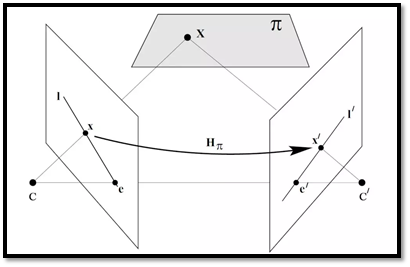图1-1 两个摄像机观察同一个场景点
所以，可以看出一个空间点在不同视角下的像点存在一种约束关系，基础矩阵F就是用来表示这种约束关系的。它是图像中的点x到另一幅图像对极线l'的映射，即l'= Fx ,而和点x匹配的另一个点x&
展开全文RANSAC
• 单目视觉SLAM可以根据其前端视觉里程计或是后端优化的具体实现算法进行分类：前端可以分为特征点法与直接法，后端可以分为基于滤波器和基于非线性优化。其中在后端上目前已经公认基于非线性优化的方法在同等计算量的...单目视觉SLAM
• 视觉里程计的任务是估计相邻图像间相机的运动，分为特征点法和直接法。...1. 特征点法 ...定义：特征点是图像当中具有代表性的部分，这些点在相机视角发生...组成：由关键点和描述部分组成 关键点——位置视觉里程计
• ## 算法-分治法-最近对问题

千次阅读 多人点赞 2019-03-11 21:23:50
用分治解决问题就是将集合s分为个集合s1和s2。每个解合都有n/2个，接着在子集和中递归求解最近的。 一维情况 为了简单化问题，我们先考虑一维情形，即所有的都在x轴上（假如顺序是有序的，也可以排好序...
• ## 轨迹优化与直接配点法

千次阅读 多人点赞 2020-04-22 01:58:00
直接配点法最终是通过非线性规划求解器来得到最终结果，因此在阅读本文之间可以先了解NLP问题相关基础知识，当然这并不影响本文的理解，本文仅需要一些简单的高等数学与微分方程的知识。 轨迹优化问题 问题简介 ...动态规划
• 特征点法VO是以提取图像中的特征点为基础，学术界有长久的研究，运行比较稳定、光照变化和动态场景鲁棒性强，是比较成熟的VO实现方案。而直接法VO是为了克服特征点法VO的部分缺点（如计算量大，不适用于纹理缺乏...
• 参考资料： 三角剖分 :https://baike.baidu.com/item/Delaunay%E4%B8%89%E8%A7%92%E5%89%96%E5%88%86%E7%AE%97%E6%B3%95?tp=2_11 TIN: ...逐插入三角剖...
• 根据伦理规范得到社会认可和被制度化的程度，我们可以把伦理规范分为制度性的伦理规范和描述性的伦理规范种情况。 正确 错误 判断题 (1/1 point) 伦理是个体性、主观性的，侧重个体的意识、行为与...
• 2D-2D: 极几何、匹配点几何关系、极约束、基础矩阵、本质矩阵、八点法、单应矩阵单应矩阵
• ## C语言

万次阅读 多人点赞 2019-12-18 23:01:50
冒泡是在扫描过程中逐次比较相邻个元素的大小。例：9+8+7+6+5+4+3+2+1=45. 9.对象间的信息传递靠消息。 10.多态性是指同一个操作可以是不同对象的行为。操作—对象。 C语言 1.源程序的扩展名为.c，目标程序的...c语言
• 论文题目：Evaluation of two architectures Using the Architecture Tradeoff ... 效用树沿着个维度排列优先顺序：每个场景系统成功的重要性以及对此场景实现（从架构师的角度来看）所带来的难度程度的估计...
• ## 测试开发笔记

万次阅读 多人点赞 2019-11-14 17:11:58
内容：需求项（业务，主要功能）需求子项，子项的详细描述 测试的工作：需求进行测试和评审A系统测试计划《系统测试计划书》B系统测试计划《系统测试方案书》C系统测试实现《系统测试用例》 ㈡设计阶段 开发经理...测试开发
• ## 学习笔记 | Heckman两阶段法介绍

万次阅读 多人点赞 2020-10-27 20:05:01
最近看的篇VC文献，都是有使用到Heckman阶段，所以就借此机会系统学习了Heckman阶段 本篇内容主要学习了如下文章： 1 CJAR的带你了解Heckman 2 计量经济圈的Heckman是什么? 及其内生性问题? 3...
• 4.下列关于牛顿法描述正确的是（） A.牛顿法是一种迭代算法，每一步都需要求解目标函数的Hessian矩阵的逆矩阵， B,二阶收敛，收敛速度快 C函数要求苛刻（二阶连续可微，汉森矩阵可逆） D.牛顿法是局部收敛的，...
• Dijkstra算法 1.定义概览 ...Dijkstra(迪杰斯特拉)算法是典型的单源最短路径算法，用于计算一个节点到其他...主要特点是以起始为中心向外层层扩展，直到扩展到终点为止。Dijkstra算法是很有代表性的最短路径算数据结构
• 在前种格式中，会将<来源>复制至<目的地>或将多个<来源>文件复制至已存在的<目录>，同时设定权限模式及所有者/所属组。在第三种格式中，会创建所有指定的目录及它们的主目录。长选项必须用的参数在使用短选项时也...
• ## 基础算法枚举法

千次阅读 2019-09-23 16:33:08
这几天刷的算法好几次都提到了枚举，虽然很早知道这个词，但是枚举的概念是迷迷糊糊的，今天特意查了一下。 枚举，也称为列举、穷举，是暴力策略的具体体现，又称为蛮力。 枚举的基本思想是: 逐一...
• 谢希仁计算机网络第七版课后答案 第一章 概述 1-01 计算机网络向用户可以提供那些...答： （1）电路交换：端端通信质量因约定了通信资源获得可靠保障，连续传送大量数据效率高。（2）报文交换：无须预约传输带...计算机网络 谢希仁
• ## 图像分割综述

万次阅读 多人点赞 2019-07-09 22:03:48
（2）相邻的个区域Ri和Rj，它们也可以大小不同（即不在同一层），如果条件H(RiURj)=TURE满足，就将它们合并起来； （3）如果进一步的分裂或合并都不可能，则结束。 其中R代表整个正方形图像区域，P代表逻辑词...图像分割
• 1、kNN算法（K 最近邻(k-Nearest Neighbors)）描述 2、KNN算法的工作原理： 3、KNN算法的一般流程 4、KNN算法的优点和缺点 5、应用KNN的常见问题 6、KNN与推荐系统 7、KNN算法的应用领域 8、KNN算法实战：...机器学习 KNN算法 Python3
• ## 测试开发需要学习的知识结构

万次阅读 多人点赞 2018-04-12 10:40:58
一些视频链接：我这有一些软件测试的视频，你可以开看看。转行互联网测试需要哪些技能？ - 假装在测试的回答 - 知乎作为一名软件测试人员，有哪些网站是你应该多多关注的，哪些书籍是你必须要看的？ - 假装在测试...测试开发
• 1、开发初期的需求定义只是用来确定软件的基本结构，使得开发初期用户只需要软件需求进行大概的描述；而对于需求的细节性描述，则可以延迟到增量构件开发时进行，以增量构件为单位逐个地进行需求补充。这种方式...软件工程
• 视觉里程计的任务是估计相邻图像间相机的运动，分为特征点法和直接法。 1. 特征点法 1.1 概念 定义：特征点是图像当中具有代表性的部分，这些点在相机视角发生微小变化后依然保持不变 组成：由关键点和描述...
• ## 精选面试自我介绍

万次阅读 多人点赞 2018-09-05 12:44:47
面试自我介绍优秀范文 （一） 各位考官好,今天能够站在这里参加面试,有机会...能关系周围的任何事,和亲人朋友能够和睦相处,并且生活充满了信心.我以前在***实习过，所以有一定的实践经验. 在外地求学的四年中，我...面试自我介绍 自我介绍
• 引言: 在深度学习的任务目标中，通常我们希望我们的学习结果能够在损失函数上得到一个较好的...首先我们知道梯度方向是函数增长最快的方向，梯度的反方向是函数减少最快的方向，而梯度下降就是往梯度反方向前进...
• 稀疏直接、定义直接的边、使用直接估计相机运动、半稠密直接、直接的讨论
• ## 层次分析法

万次阅读 2015-06-11 18:11:53
层次分析（Analytic Hierarchy Process，简称AHP）是将与决策总是有关的元素分解成目标、准则、方案等层次，在此基础之上进行定性和定量分析的决策方法。该方法是美国运筹学家匹茨堡大学教授萨蒂于20世纪70年代初......